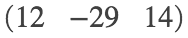# MATH 251: Calculus 3, SET8

## 13: Vectors Functions

### 13.2: Derivatives and Integrals of Vector Functions

These problems are done with the CAS. See Hand Solutions for details.

#### 1. [860/4]

A parametric curve is plotted in the xy-plane. Its position and velocity vectors at a point are shown.
syms t
r(t) = [t^2 t^3], Dr(t) = diff(r(t), t) % plane curve
r(t) =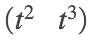Dr(t) =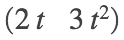r1 = r(1), v1 = Dr(1)
r1 =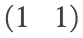v1 =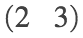figure
fplot(t^2, t^3, [0, 1.6], 'm', 'LineWidth', 4); grid on; hold on
plot(1, 1, 'go', 'MarkerFaceColor', 'g', 'MarkerSize', 10)
quiver(0,0, 1,1, 'b', 'LineWidth', 2, 'AutoScale', 'off')
quiver(1,1, 2,3, 'r', 'LineWidth', 2, 'AutoScale', 'off')
axis equal; axis([0 3 0 4])
xlabel('x'); ylabel('y')
title('SET8, 860/4')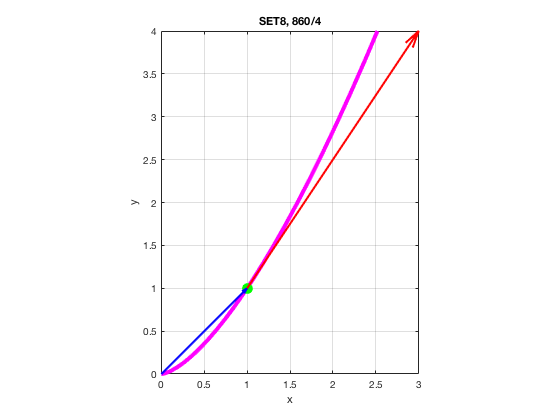#### 2. [860/10]

The derivative of a parametric space curve is computed.
syms t
r = [exp(-t) t-t^3 log(t)]
r =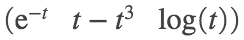Dr = diff(r, t)
Dr =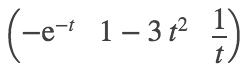#### 3. [860/14]

The derivative of another parametric space curve is computed.
syms a b c t
r = [sin(a*t)^2 t*exp(b*t) cos(c*t)^2]
r =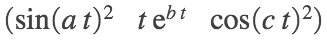Dr = diff(r, t)
Dr =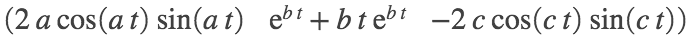Dr_simplified = simplify(Dr)
Dr_simplified =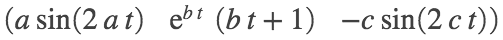#### 4. [860/20]

A unit tangent vector at a point along a space curve is determined.
syms t
r(t) = [sin(t)^2 cos(t)^2 tan(t)^2], Dr(t) = diff(r(t), t)
r(t) =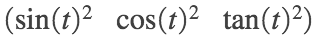Dr(t) =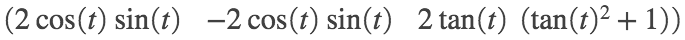v = Dr(pi/4) % tangent vector
v =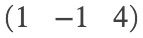T = v/norm(v) % UNIT tangent vector
T =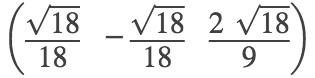len_T = norm(T)
len_T = 1

#### 5. [860/26]

A space curve and its tangent line at a point are illustrated.
syms t u
r(t) = [sqrt(t^2 + 3) log(t^2 + 3) t] % space curve
r(t) =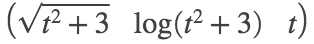Dr(t) = diff(r(t), t)
Dr(t) =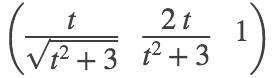r1 = r(1), v1 = Dr(1)
r1 =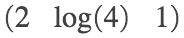v1 =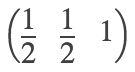L(t) = r1 + t*v1 % tangent line
L(t) =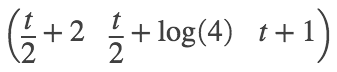figure
fplot3(sqrt(t^2 + 3), log(t^2 + 3), t, [0, 2], 'LineWidth', 4); grid on; hold on
fplot3(2+u/2, log(4)+u/2, u+1, [-1 1], 'LineWidth', 4); grid on; hold on
plot3(2, log(4), 1, 'go', 'MarkerFaceColor', 'g', 'MarkerSize', 10)
axis equal; axis([1.5 3.0 0.5 2.0 0.0 2.0])
xlabel('x'); ylabel('y'); zlabel('z')
xticks(1.5:0.5:3.0); yticks([0.5:0.5:2.0]); zticks(0.0:0.5:2.0)
title('SET8, 860/26')
view(44,13)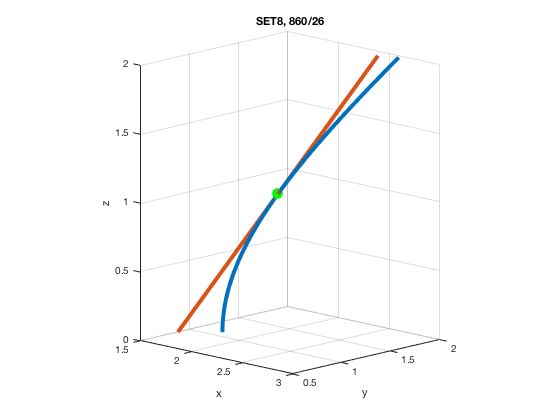#### 6. [860/30]

Another space curve and its tangent line at a point are illustrated.
syms t u
r(t) = [2*cos(t) 2*sin(t) 4*cos(2*t)] % space curve
r(t) =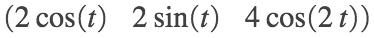Dr(t) = diff(r(t), t), tp = sym(pi/6), s = sym(sqrt(3))
Dr(t) =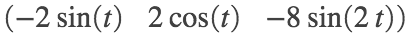tp =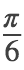s =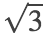rp = r(tp), vp = Dr(tp)
rp =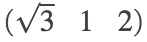vp =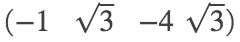L(t) = rp + t*vp % tangent line
L(t) =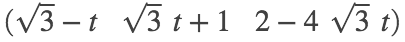figure
fplot3(2*cos(t), 2*sin(t), 4*cos(2*t), [0, 2*pi], 'LineWidth', 4); grid on; hold on
fplot3(s-u, s*u+1, 2-4*s*u, [-1 1], 'LineWidth', 4); grid on; hold on
plot3(s, 1, 2, 'go', 'MarkerFaceColor', 'g', 'MarkerSize', 10)
xlabel('x'); ylabel('y'); zlabel('z')
title('SET8, 860/30')
view(44,13)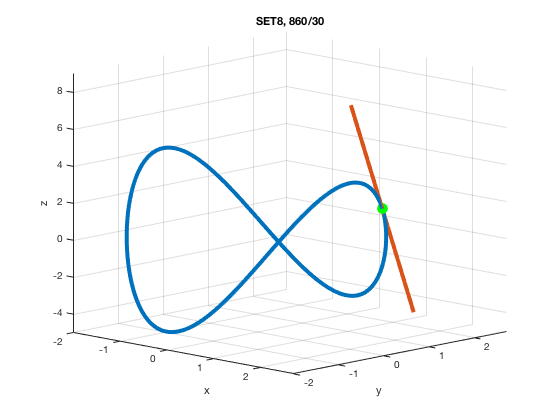#### 7. [861/34]

The angle of intersection of two space curves is calculated.
syms s t
r1(t) = [t 1-t 3+t^2], r2(s) = [3-s s-2 s^2]
r1(t) =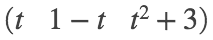r2(s) =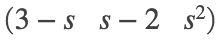Dr1(t) = diff(r1(t), t), Dr2(s) = diff(r2(s), s)
Dr1(t) =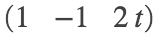Dr2(s) =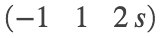eq = r1(t) == r2(s)
eq =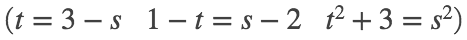soln = solve(eq, [s t]); si = soln.s, ti = soln.t % parameter values at intersection
si = 2
ti = 1
P = r1(ti), P = r2(si) % intersection point
P =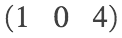P =v1 = Dr1(ti), v2 = Dr2(si)
v1 =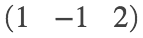v2 =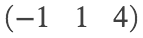theta = round(double(acosd(dot(v1,v2) / (norm(v1)*norm(v2)))), 0)
theta = 55
% The angle of intersection is 55 degrees (to the nearest degree).
% Here is an illustration.
figure
fplot3(t, 1-t, 3+t^2, [0, 2], 'b', 'LineWidth', 2); grid on; hold on
fplot3(3-s, s-2, s^2, [1 3], 'r', 'LineWidth', 2); grid on; hold on
plot3(1, 0, 4, 'go', 'MarkerFaceColor', 'g', 'MarkerSize', 10)
quiver3(1,0,4, 1,-1,2, 'c', 'LineWidth', 1, 'AutoScale', 'off')
quiver3(1,0,4, -1,1,4, 'm', 'LineWidth', 1, 'AutoScale', 'off')
axis equal; axis([-1 3 -2 2 1 9])
xlabel('x'); ylabel('y'); zlabel('z')
title('SET8, 860/34')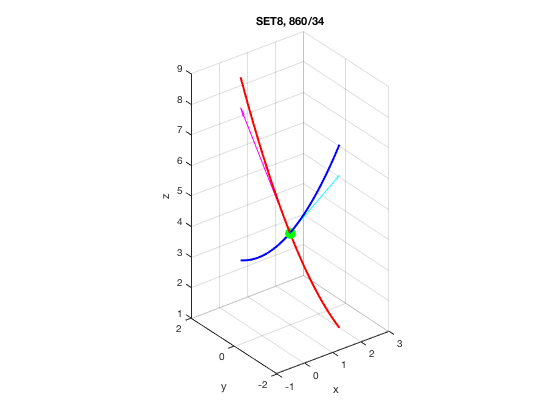#### 8. [861/36]

A vector integral is computed.
syms t
r = [2*t^(3/2) 0 (t+1)*sqrt(t)]
r =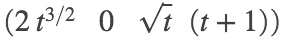I = int(r, t, 1, 4)
I =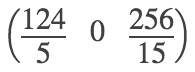I_appx = double(I)
I_appx = 1×3
24.8000 0 17.0667

#### 9. [861/42]

A position function is found from its derivative (velocity) and its initial position.
syms C1 C2 C3 t
C = [C1 C2 C3]
C =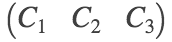Dr(t) = [t exp(t) t*exp(t)]
Dr(t) =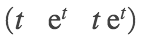r(t) = int(Dr(t), t) + C
r(t) =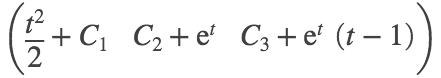eqs = r(0) == [1 1 1]
eqs =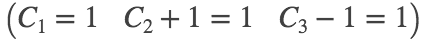s = solve(eqs, C), K1 = s.C1, K2 = s.C2, K3 = s.C3
s = struct with fields:
C1: [1×1 sym] C2: [1×1 sym] C3: [1×1 sym]
K1 = 1
K2 = 0
K3 = 2
K = [K1 K2 K3]
K =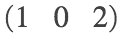solution = subs(r(t), C, K)
solution =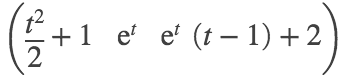#### 10. [861/50]

We have r'(t) = (u(t) x v(t))' = u'(t) x v(t) + u(t) x v'(t). Thus r'(2) = u'(2) x v(2) + u(2) x v'(2).
We are given u(2) = [1 2 -1], u'(2) = [3 0 4], and v(t) =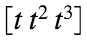. So v'(t) =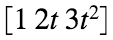. Now plug in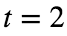. See below.
u2 = sym([1 2 -1]), Du2 = sym([3 0 4])
u2 =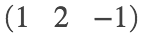Du2 =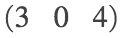v2 = sym([2 4 8]), Dv2 = sym([1 4 12])
v2 =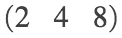Dv2 =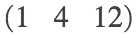Dr2 = cross(Du2,v2) + cross(u2,Dv2)
Dr2 =